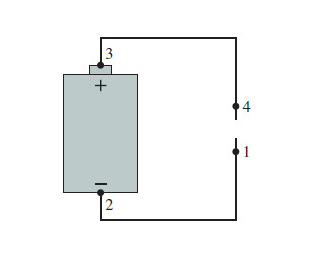# Problem: 5 V battery with metal wires attached to each end.What are the potential differences ΔV12=V2−V1, ΔV23=V3−V2, ΔV34=V4−V3, and ΔV41=V1−V4?

###### FREE Expert Solution

The potential at points 1 and 2 is the same.

V1 = V2

ΔV12 = 0 V

85% (498 ratings)###### Problem Details

5 V battery with metal wires attached to each end.What are the potential differences ΔV12=V2−V1, ΔV23=V3−V2, ΔV34=V4−V3, and ΔV41=V1−V4?

Frequently Asked Questions

What scientific concept do you need to know in order to solve this problem?

Our tutors have indicated that to solve this problem you will need to apply the Electric Potential concept. You can view video lessons to learn Electric Potential. Or if you need more Electric Potential practice, you can also practice Electric Potential practice problems.

What professor is this problem relevant for?

Based on our data, we think this problem is relevant for Professor Costantino's class at PSU.